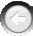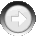﻿ SetDiversity

# SetDiversity

Navigation:  »No topics above this level«

# SetDiversitySetDiversity(Population As Integer, DiversityRate As Single, DiversityRadius As Single)   Purpose:  This function sets new parameters of the additional genetic algorithm operator called Diversity.  This operator is applied to continuous (noninteger and integer) chromosomes only, and does not apply to enumerated chromosomes. Diversity is a kind of non-uniform mutation:  it helps to investigate, with high speed, the local space around current values of the problem parameters to be optimized.  By default, the diversity operator is off in the DLL, so both DiversityRate and DiversityRadius are set to zero.  To activate the operator you will need to set these operator parameters to some non-zero values.   Remember that the Diversity operator may only be applied to individual continuous chromosomes created by the MakeChromosome function. Diversity cannot be applied to a chromosome pool created by the MakeChromosomePool function.  The Diversity operator is automatically applied in the DLL if DiversityRate and DiversityRadius both are set to non-zero values by the SetDiversity function.   Arguments: Population is the number of the population for which you are going to set the parameters of the operators. DiversityRate defines the probability that the diversity operator is applied to a chromosome.  Allowed values are in the range [0,1] and recommended values are 0.1-0.5.  Default value is 0. DiversityRadius is the radius of the area where the diversity operator is active.  Allowed values are from the range [0,1], where DiversityRadius = 1 corresponds to the effective radius of Abs(MaxValue-MinValue) on the scale of each problem parameter.  The default value is 0.   Example: i = SetDiversity(PopNum, 0.1, 0.001)   This example sets parameters of the diversity operator for the population PopNum.  The diversity rate will be 0.1, and the diversity radius will be 0.001.   Related functions: none